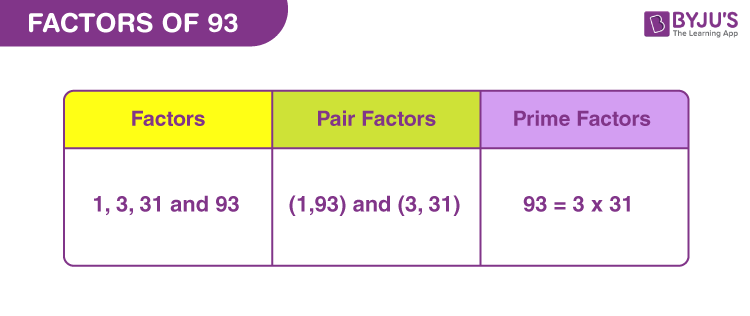# Factors of 93

In Mathematics, factors of 93 are those real numbers that completely divide the original number. 93 is a composite number and has four factors. These factors are determined by the division method. 93 is the third multiple of 31. Since, 31 x 3 = 93.

Now, let us find what are the numbers that are the factors of 93 along with its pair factors and prime factors.## How to Find Factors of 93?

Factors of 93 are the integers that divide the original number, evenly or equally.

By dividing 93 by the sequence of natural numbers we can determine which are the required factors. If the quotient after division is a whole number, then the divisor is the factor.

93 ÷ 1 = 93

93 ÷ 3 = 31

93 ÷ 31 = 3

93 ÷ 93 = 1

Thus, the factors of 93 are 1, 3, 31 and 93.

## Pair Factors of 93

The product of those factors that result in the original number is called pair factors. Thus, the product of pair factors of 93 will result in 93.

1 × 93 = 93

3 × 31 = 93

Therefore, the pair factors are (1, 93) and (3, 31).

Similarly, considering the negative pair factors, we get;

-1 × -93 = 93

-3 × -31 = 93

Therefore, the negative pair factors are (-1, -93) and (-3, -31).

## Prime Factorisation of 93

Prime factors of 93 are the prime numbers that can divide the original number into equal parts. We can use the prime factorization method to find the prime factors of a number. In this method, we need to divide 93, each time, by a prime number unless we get 1.

List of prime numbers (1 to 100)

 2, 3, 5, 7, 11, 13, 17, 19, 23, 29, 31, 37, 41, 43, 47, 53, 59, 61, 67, 71, 73, 79, 83, 89, 97

Step 1: Since 93 is an odd number, we cannot divide it by 2. 93 divided by 3.

93/3 = 31

Step 2: Now 31 is itself a prime number, as we can see from the list. Thus,

31/31 = 1

Therefore,

 Prime factorisation of 93 = 3 x 31

## Solved Examples

Q.1: If 93 is divided by 31, then in how many parts is it divided?

Solution: Given,

93 divided by 31 = 93/31 = 3

Therefore, 93 is divided into 3 equal parts.

Q.2: Find the sum of all the factors of ninety-three.

Solution: The factors of 93 are 1, 3, 31 and 93.

Sum = 1 + 3 + 31 + 93 = 128

Therefore, 128 is the required sum.

Q.3: What is the greatest common factor of 63 and 93?

Answer: Let us write the factors of both the numbers.

63 → 1, 3, 7, 9, 21, 63

93 → 1, 3, 31, 93

Hence, the greatest common factor is 3.

## Frequently Asked Questions on Factors of 93

### 1. What are the factors of 93?

Answer: There are a total of four factors of 93. These factors are 1, 3, 31 and 93.

### 2. What are the multiples of 93?

Answer: The first 10 multiples of 93 are 93, 186, 279, 372, 465, 558, 651, 744, 837 and 930.

### 3. Is 4 a factor of 93?

Answer: No, 4 is not a factor of 93. 93 divided by 4 produces a fraction and a fraction cannot be a factor.

93/4 = 23.25

### 4. Is 93 a prime number?

Answer: 93 is not a prime number but a composite number because it has more than 2 factors.

### 5. What is 93 as a product of primes?

Answer: The prime factorisation of 93 is:

93 = 3 x 31

### 6. What is the mean of factors of 93?

Average = (1 + 3 + 31 + 93)/4

Average = 128/4 = 32

Therefore, 32 is the required mean.